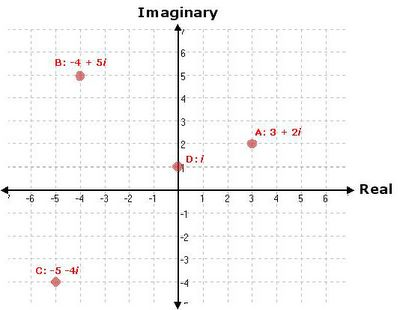Jun 9, 2005

part 1 - imaginary numbers

I thought I'd do a piece on imaginary numbers. When I was in grad school I took an entire class on imaginary numbers. The professor was enraptured with them. And over the course of the class I realized why. They are seemingly nonsensical things but are absolutley necessary to really do the full range of mathematics that is required for physics. In fact the deeper you get into physics the more you realize how intrinsically non-imaginary they really are. I started reading The Road to Reality and much of the first section is taken up by imaginary number theory. I realized how much I had forgotten. Anyway here are my notes.

Most people are somewhat aware of imaginary numbers. Most people seem to know it through the equation,
e(i π) + 1 = 0
e is a number. i is the imaginary number, and π is Pi. 5 of the most basic numbers of mathematics all rolled up into one equation. Elegant. Much of imaginary number theory is like this. So what is "i" here,
i = sqrt(-1) = (-1)(1/2)
Now it might seem funny to us that we even need this or that such a quantity can exist or have any meaning or use in the world of mathematics much less outside it. But it turns out that we do need them. In fact the deeper you go into physics the more they seem to pop up. You didn't need them in high school physics at all. But to do quantum mechanics "i" is everywhere.

Since you have all these numbers you realize there are rules governing how they interact. You can add, subtract, multiply, raise to another integer, square root, etc. You take a look at the operation sqrt(2). Looks straightforward. Let me figure out what rational number is the answer to that. Turns out there isn't one. You seem to get close but you can never take a finite integer divided by another finite integer and get the number that equals sqrt(2). Turns out you need some more numbers - irrational numbers. It's a bad name. You realize these numbers have some unique properties. If I take my rational numbers and write them in decimal form they start repeating after a while.
1/2 = 0.55555555...
1/10 = 0.1000000...
1/6 = 0.1666666...
Irrational numbers don't ever seem to repeat. Fair enough. We clearly need to use the sqrt(2) and Pi so let's add them to our list of numbers. Now you look at sqrt(-1). Again you can't find a number in your list that solves this equation. So you make a supposition. Let me call the answer to this "i". And let me assume that all I can write imaginary numbers as such,
a + bi
where a and b are just constants. 'a' is the real number component and 'b' is the imaginary component. If a + bi is truly a number then I should be able to perform all the types of mathematical operations on them that I'm used to doing on my other numbers - add, subtract, multiply, divide, raise to a power of, take a cosine of it, etc. And the result of that operation should be of the form a + bi (except the a and b will be different after the operation). Turns out this is the case. It's a little more involved than the way we normally do those operations but not horribly different. These imaginary numbers behave like all our other numbers.

So the point I'm trying to make here is that our addition of 'i' is no stranger than our addition of irrationals. Irrationals really are just as hard to get your head around. We can't write them out in fraction or decimal form. They can only be truly represented by symbols (Pi) or by operations on other rationals; sqrt(2). So they are really just as elusive to our understanding. We can't internalize an irrational any more than an imaginary number. It's just that we've dealt with irrationals since grade school so they seem a little more 'real'. Now how do we go about visualizing "i"? The easiest way is to effectively consider the real and imaginary components of the a + bi as separate 'dimensions' and plot numbers out on a plane. Check out the graph below.the x-axis is the real component and the y-axis is the imaginary component. You can see some imaginary numbers plotted on it. As a result of our choice they effectively behave like 2-D vectors but we shouldn't make the jump to thinking of imaginary numbers as vectors. It's just a representation to help us think about it.

Next we'll do some mathematical operations that show how adding this imaginary component to our list of numbers we can use can help us understand non-imaginary number functions to a much deeper degree even when imaginary numbers are not really part of the function or the answer.